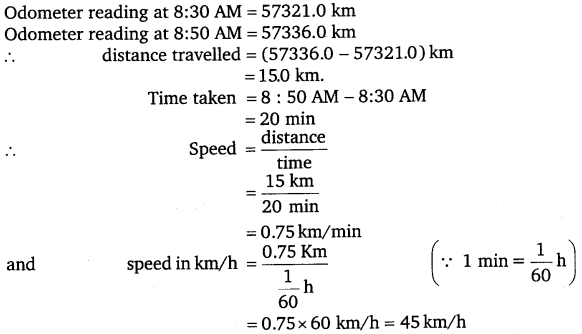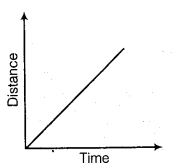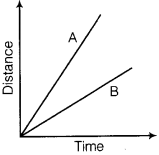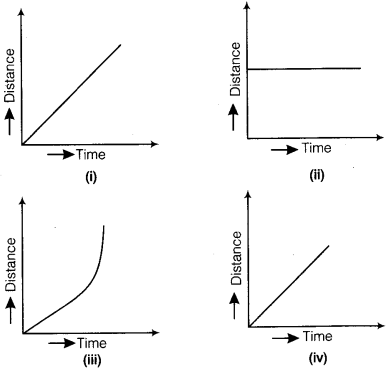# Class 7 Science NCERT Solutions for Chapter – 13 Motion and Time

## Motion and Time

Question 1.
Classify the following as motion along a straight line, circular or oscillatory motion :

1. Motion of your hands while running.
2. Motion of a horse pulling a cart on a straight road.
3. Motion of a child in a merry-go-round.
4. Motion of a child on a see-saw.
5. Motion of the hammer of an electric bell.
6. Motion of a train on a straight bridge.

Solution:

1. oscillatory
2. straight line
3. circular
4. oscillatory
5. oscillatory
6.  straight line.

Question 2.
Which of the following are not correct?
(i)
The basic unit of time is second.
(ii) Every object moves with a constant speed.
(iii) Distances between two cities are measured in kilometres.
(iv) The time period of a given pendulum is not constant.
(v) The speed of a train is expressed in m/h.

Solution:
(ii) , (v).

Question 3.
A simple pendulum takes 32 s to complete 20 oscillations. What is the time period of the pendulum?

Solution:
Time taken for 20 oscillations = 32 s
Time taken for 1 oscillation =$\frac { 32 s }{ 20 }$
Hence,   Time period = 1.6 s

Question 4.
The distance between two stations is 240 km. A train takes 4 hours to cover this distance. Calculate the speed of the train.

Solution :
Given, distance = 240 km,
time = 4 h,
speed =$\frac { distance }{ time }$ =$\frac { 240 km }{ 4 h }$ = 60 km/h.

Question 5.
The odometer of a car reads 57321.0 km when the clock shows the time 08 : 30 AM. What is the distance moved by the car, if at 08 : 50 AM, the odometer reading has changed to 57336.0 km? Calculate the speed of the car in km/min during this time. Express the speed in km/h also.

Solution:Question 6.
Salma takes 15 minutes from her house to reach her school on a bicycle. If the bicycle has a speed of 2 m/s, calculate the distance between her house and the school.

Solution:
Given, Speed = 2 m/s
Time =15 min = 15 x 60 s = 900 s
distance = speed x time
= 2 m/s x 900 s = 1800 m$\frac { 1800 m }{ 1000 }$ km
= 1.8 km

Question 7.
Show the shape of the distance-time graph for the motion in the following cases :
(i)
A car moving with a constant speed.
(ii) A car parked on a side road.

Solution:
(i) A car moving with a constant speed covers equal distance in equal intervals of time(ii) The distance-time graph of a car parked on a road side is such that with the increase in time, there is no change in distance.Question 8.
Which of the following relations is correct?
(i)
Speed = Distance x Time
(ii) Speed =$\frac { distance }{ time }$
(iii) Speed =$\frac {time }{ distance }$
(iv) Speed =$\frac { 1 }{ Distance x Time }$

Solution:
(ii) Speed =$\frac { distance }{ time }$

Question 9.
The basic unit of speed is :
(i) km/min
(ii) m/min
(iii) km/h
(iv) m/s

Solution:
(iv) m/s

Question 10.
A car moves with a speed of 40 km/h for 15 minutes and then with a speed of 60 km/h for the next 15 minutes. The total distance covered by the car is:
(i)
100 km
(ii) 25 km
(iii) 15 km
(iv) 10 km

Solution:
Distance travelled in first 15 min
= speed x time
= 40 km/h x 15 min
= 40 km/h x 15/60 h = 10 km
Distance travelled in last 15 min
= speed x time
= 60 km/h x 15 min
= 60 km/h x 15/60 h = 15 km
Total distance = (10 +15) km = 25 km
Hence, option (ii) is correct.

Question 11.
Suppose the two photographs, shown in Fig. 13.1 and Fig. 13.2, had been taken at an interval of 10 seconds. If a distance of 100 metres is shown by 1 cm
in these photographs, calculate the speed of the blue car.

Solution:
Speed = 100 m/10 s = 10 m/s

Question 12.
shows the distance-time graph for the motion of two vehicles A and B. Which one of them is moving faster?Solution:
Vehicle A is moving faster.

Question 13.
Which of the following distance-time graphs shows a truck moving with speed which is not constant?Solution:
Graph (iii).